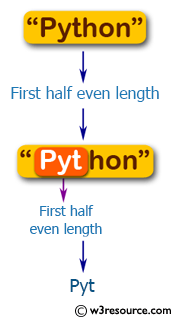﻿ Swift String Programming Exercise: Return the first half of a given string of even length - w3resource# Swift String Exercises: Return the first half of a given string of even length

## Swift String Programming: Exercise-5 with Solution

Write a Swift program to return the first half of a given string of even length.

Pictorial Presentation:Sample Solution:

Swift Code:

``````func first_half_str(_ input: String) -> String {
let chars = input.characters
let first_half = chars.prefix(input.characters.count / 2)

return String(first_half)
}
print(first_half_str("Java"))
print(first_half_str("Python"))
```
```

Sample Output:

```
Ja
Pyt
```

Swift Programming Code Editor:

Improve this sample solution and post your code through Disqus

What is the difficulty level of this exercise?

﻿